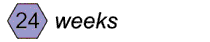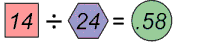Short-Term Goal Calculator
To calculate the short-term goal for a student, enter the following, then click the “Calculate” button.
End of year benchmark

Average of the scores on the first three probes

Number of weeks left in the school year

Number of weeks the student is being monitored

View Directions

#### Short-Term Goal Calculation – DirectionsTo determine the short-term goal, the teacher must have the following pieces of information in hand:

• The end-of-year benchmark
• The student’s average CBM score on the first three probes
• The number of weeks left in the school year
• The number of weeks of monitoring

The table below outlines step-by-step directions for calculating a short-term goal.

Calculation Directions Example
Step 1 Determine the minimum number of words that the student needs to gain across the year.
• Start with the end-of-year benchmark for a given grade level (e.g., 30 words correct per minute [wcpm]).
• Subtract the student’s average score on the first three CBM probes (e.g., 16 wcpm).

Example:Step 2 Determine the number of weeks left in the school year. Example:Step 3 Determine the minimum number of words that the student needs to achieve each week in order to meet the end-of-year benchmark. Divide the minimum number of words that the student needs to gain across the year by the number of weeks left in the school year.

Example:Step 4 Determine the word gain, that is how many more words the student should be able to read at the end of the monitoring period. Multiply the weekly gain (.58) by the number of weeks the student is monitored (e.g., 10). This process indicates the number of words the student needs to gain by the end of the monitoring period.

Example:After 10 weeks of monitoring, the student needs to read 6 more words than he or she was reading at the beginning of the monitoring period.

Step 5 Determine the short-term goal. Add the short-term gain (6) to the student’s initial score (16 wcpm).

Example:At the end of 10 weeks of progress monitoring, the student should be reading 22 wcpm.

(Close these directions)